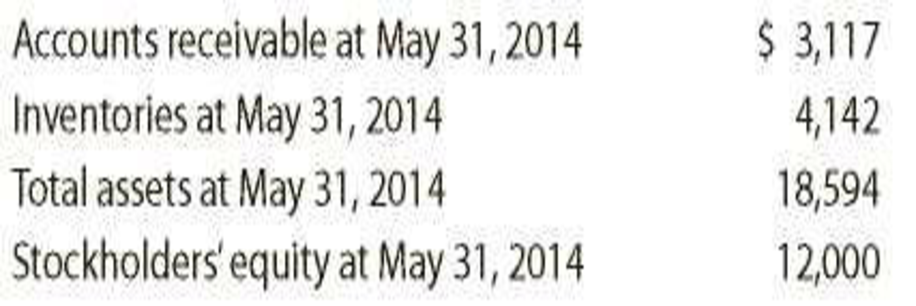Chapter 17, Problem 1FSA

Chapter
Section
Textbook Problem
1 views

# Financial statement analysisThe financial statements for Nike, Inc., are presented in Appendix D at the end of the text. Use the following additional information (in thousands):Instructions 1. Determine the following measures for the fiscal years ended May 31, 2016, and May 31, 2015. Round ratios and percentages to one decimal place. a. Working capital b. Current ratio c. Quick ratio d. Accounts receivable turnover e. Number of days’ sales in receivables f. Inventory turnover g. Number of days’ sales in inventory h. Ratio of liabilities to stockholders’ equity i. Asset turnover j. Return on total assets. k. Return on common stockholders’ equity l. Price-earnings ratio, assuming that the market price was $54.90 per share on May 29, 2016, and$52.81 per share on May 30, 2015 m. Percentage relationship of net income to sales 2. What conclusions can be drawn from these analyses?

1.

To determine

Determine the following ratios for the fiscal years ended May 31, 2016 and May 31, 2015:

1. (a) Working capital
2. (b) Current ratio
3. (c) Quick ratio
4. (d) Accounts receivable turnover ratio
5. (e) Number of days’ sales in receivables
6. (f) Inventory turnover ratio
7. (g) Number of days’ sales in inventory
8. (h) Ratio of liabilities to stockholders’ equity
9. (i) Asset turnover ratio
10. (j) Return on total assets
11. (k) Return on common stockholders’ equity
12. (l) Price-earnings ratio
13. (m) Percentage relationship of net income  to sales
Explanation

Financial Ratios: Financial ratios are the metrics used to evaluate the liquidity, capabilities, profitability, and overall performance of a company.

(a)

Working capital is determined as the difference between current assets and current liabilities.

Formula:

Working capital = Current assets – Current liabilities

Calculate working capital for May 31, 2015 and May 31, 2016.

Working capital  (2016)= Current assets – Current liabilities = $15,025.0 –$5,358.0= $9,667.0 Working capital (2015)= Current assets – Current liabilities =$15,587.0 – $6,332.0=$9,255.0

Thus, working capital for 2016 and 2015 is $9,667.0 and$9,255.0

b)

Current ratio is used to determine the relationship between current assets and current liabilities. The ideal current ratio is 2:1.  Current assets include cash and cash equivalents, short-term investments, net, accounts and notes receivables, net, inventories, and prepaid expenses and other current assets. Current liabilities include short-term obligations and accounts payable.

Formula:

Current ratio=Current assetsCurrentliabilities

Current ratio for 2016 and 2015

Current ratio(2016)=Current assetsCurrentliabilities=$15,025.0$5,358.0=2.8

Current ratio(2015)=Current assetsCurrentliabilities=$15,587.0$63,320=2.5

Thus, current ratio for 2016 and 2015 is 2.8 and 2.5

c)

Acid-Test Ratio is the ratio denotes that this ratio is a more rigorous test of solvency than the current ratio. It is determined by dividing quick assets and current liabilities. The acceptable acid-test ratio is 0.90 to 1.00. Use the following formula to determine the acid-test ratio:

Acid Ratio=Quick assetsCurrentliabilities

Acid-test ratio for 2016

Acid-test ratio (2016)=Quick assets Currentliabilities=$8,698.0$5,358.0=1.6

Acid-test ratio (2015)=Quick assets Currentliabilities=$9,282.0$6,332.0=1.5

Working notes:

Quick Assets are those assets that are most liquid. The examples of quick assets include cash and bank balances, marketable securities, and sundry debtors. Use the following formula to determine the Quick assets:

Quick assets  for 2016=(Cash + Short-term investments + Net accounts and notes receivables)=$3,138.0+$2,319.0+$3,241.0=$8,698.0

Quick assets  for 2015=(Cash + Short-term investments + Net accounts and notes receivables)=$3,852.0+$2,072.0+$3,358.0=$9,282.0

Hence, quick ratio for 2016 and 2015 are 1.6 and 1.5 respectively.

d)

Accounts receivable turnover ratio for 2016 and 2015

Accounts receivables turnover ratio (2016)}=Net credit salesAverage accounts receivables=$32,376.0$3,299.5=9.8

Accounts receivables turnover ratio (2015)}=Net credit salesAverage accounts receivables=$30,601.0$3,237.5=9.5

Description:

Accounts receivables turnover ratio is mainly used to evaluate the collection process efficiency. It helps the company to know the number of times the accounts receivable is collected in a particular time period. Main purpose of accounts receivable turnover ratio is to manage the working capital of the company. This ratio is determined by dividing credit sales and sales return.

Formula:

Accounts receivables turnover ratio}=Net credit salesAverage accounts receivables

Average accounts receivable, net for 2016 and 2015 is determined as follows:

Average accounts receivables (2016)}(Opening accounts receivables + Closing accounts receivables )2=$3,358.0+$3,241.02=$3,299.50 Average accounts receivables (2015)}(Opening accounts receivables + Closing accounts receivables )2=$3,117.0+$3,358.02=$3,237.50

Hence, the accounts receivable turnover ratio for 2016 and 2015 is 9.8 times and 9.5 times.

e)

Number of days’ sales in receivables for 2016 and 2015

Number of days’ sales in receivable (2016)}=Average accounts receivable Average daily sales=$3,299.5088.7=37.2days Number of days’ sales in receivable (2015)}=Average accounts receivable Average daily sales=$3,237.5083.8=38.6days

Description:

Number of days’ sales in receivables is used to determine the number of days a particular company takes to collect accounts receivables.

Formula:

Number of days’ sales in receivable=Average accounts receivable Average daily sales

Average daily sales are determined by dividing sales by 365 days. Average accounts receivables for 2016 and 2015 are determined in previous requirement. Thus, average daily sales for 2016 and 2015 are determined as follows:

Average daily sales (2016)Sales365days=$32,376.0365days=$88.7

Average daily sales (2015)Sales365days=$30,601.0365days=$83.8

Hence, the number of days’ sales in receivable for 2016 and 2015 are 9.8 days and 9.5 days respectively.

f)

Inventory turnover ratio for 2016 and 2015

Inventory turnover ratio (2016)=Cost of goods soldAverage inventory=$17,405.0$4,587.5=3.8times

Inventory turnover ratio (2015)=Cost of goods soldAverage inventory=$16,534.0$4,142.0=4.0times

Description:

Inventory turnover ratio is used to determine the number of times inventory used or sold during the particular accounting period.

Formula:

Inventory turnover=Cost of goods soldAverage inventory

Average inventory for 2016 and 2015 is determined as below:

Average inventory (2016)}(Opening inventory + Closing inventory )2=$4,337.0+$4,838.02=$4,587.50 Average inventory (2015)}(Opening inventory + Closing inventory )2=$3,947.0+$4,337.02=$4,142.0

Hence, the inventory turnover ratio for 2016and 2015 is 3

2.

To determine

### Still sussing out bartleby?

Check out a sample textbook solution.

See a sample solution

#### The Solution to Your Study Problems

Bartleby provides explanations to thousands of textbook problems written by our experts, many with advanced degrees!

Get Started

#### Find more solutions based on key concepts